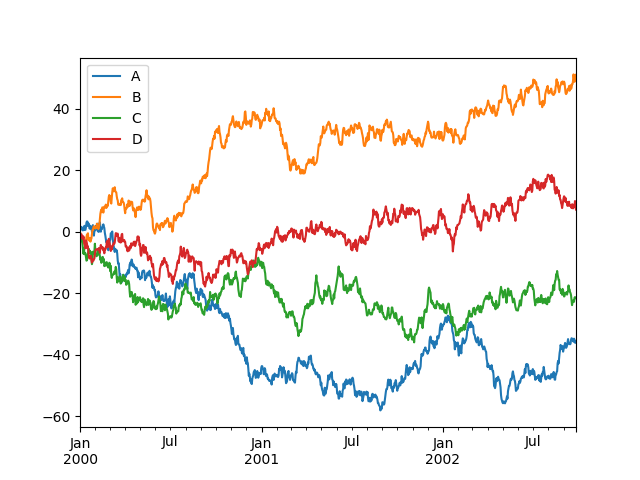# Plotting With Pandas

Last updated: May 14th, 2019# Plotting with Pandas¶

The pandas library has become popular not just for enabling powerful data analysis, but also for its handy pre-canned plotting methods. Interestingly though, pandas plotting methods are really just convenient wrappers around existing matplotlib calls.

That is, the plot() method on pandas’ Series and DataFrame is a wrapper around plt.plot() we'll see in upcoming lectures.## Hands on!¶

In [ ]:
import numpy as np
import pandas as pd


Pandas can easily read data stored in different file formats like CSV, JSON, XML or even Excel as we saw on the previous lecture.

Let's read some data and plot some basic figures.

In [ ]:
# load data
df = pd.read_csv('data/nba_data.csv')

# show first rows
df.head()### Plotting basics¶

Let's see some common used type of plots:

In [ ]:
df.plot?### Scatter plot¶

We'll use Fg and Fga to draw a scatter plot and see the relation between both columns.

In [ ]:
df.plot(kind='scatter',
x='Fg',
y='Fga',
color='orange')

In [ ]:
df.plot.scatter(x='Fg',
y='Fga',
color='orange')


Now let's see relation between Fg and Age:

In [ ]:
df.plot(kind='scatter',
x='Fg',
y='Age',
color='purple')

In [ ]:
df.plot(kind='scatter',
x='Fg',
y='Age',
color='purple',
s=df['Fga']*5)### Bar plot¶

We can also make some nice bar plots. Let's see the age of the first 10 players:

In [ ]:
df.head(10).plot(kind='bar',
x='Player',
y='Age')

In [ ]:
df.head(10).plot.bar(x='Player',
y='Age')

In [ ]:
df.head(10)['Age'].plot(kind='bar')


Also, we can show multiple columns in the same plot:

In [ ]:
df.head(10)[['Fg', 'Fga']].plot(kind='bar')### Line plot¶

Another useful type of plot is the line plot.

In [ ]:
df.head(10).plot(kind='line',
x='Player',
y='Age')### Pie plot¶

In order to see the fractional area of different values within a column, we can make a pie plot.

In [ ]:
df.head(5)['Fg'].plot(kind='pie',
y='Fg')

In [ ]:
df.head(5)['Fg'].plot(kind='pie',
y='Fg',
title='FG per Player',
labels=df['Player'],
figsize=(6,6))

In [ ]:
df.head(5)['Fg'].plot(kind='pie',
y='Fg',
labels=df['Player'],
title='FG per Player',
legend=True,
figsize=(6,6))### Histogram¶

Histograms can also be drawn.

In [ ]:
df['Age'].plot(kind='hist',
rwidth=0.85)

In [ ]:
df['Age'].plot(kind='hist',
rwidth=0.85,
orientation='horizontal')### KDE plot¶

We can also generate Kernel Density Estimate plot (KDE) using Gaussian kernels.

In statistics, kernel density estimation (KDE) is a non-parametric way to estimate the probability density function (PDF) of a random variable. This function uses Gaussian kernels and includes automatic bandwidth determination.

In [ ]:
df['Age'].plot(kind='kde')

In [ ]:
df[['Fg', 'Fga']].plot(kind='kde')### Box plot¶

Box plot can also be drawn.

In [ ]:
df.head(10)[['Fg', 'Fga']].plot(kind='box')

In [ ]:
df.head(10)[['Age']].plot(kind='box')

In [ ]:
df.head(10)[['Age']].plot.box()

In [ ]:
df.head(10)[['Age', 'Position']].boxplot(by='Position')### Dive into a real world example¶

We'll introduce another useful library called matplotlib.

Matplotlib is a Python 2D plotting library which produces publication quality figures in a variety of hardcopy formats and interactive environments across platforms. Matplotlib can be used in Python scripts, the Python and IPython shells, the Jupyter notebook, web application servers, and four graphical user interface toolkits.

To let matplotlib render graphics inside a Notebook, we should execute %matplotlib inline.

In [ ]:
import matplotlib.pyplot as plt

%matplotlib inline


Pandas can easily read data stored in different file formats like CSV, JSON, XML or even Excel as we saw on the previous lecture. Let's read some data and plot some basic figures.

In [ ]:
# load data
df = pd.read_csv('data/btc-market-price.csv',
parse_dates=,
header=None)

# add column names
df.columns = ['Timestamp', 'Price']

# define 'Timestamp' as index
df.set_index('Timestamp', inplace=True)

# show first rows
df.head()


#### Histograms¶

The hist() function automatically generates histograms and returns the bin counts or probabilities:

In [ ]:
plt.hist(df['Price'])


plt.plot() accepts many parameters, but the first two ones are the most important ones: the values for the X and Y axes.

Let's make a plot showing $f(x) = x^2$:

In [ ]:
x_values = np.arange(10)
y_values = [value ** 2 for value in x_values]

plt.plot(x_values, y_values)

In [ ]:
x_values = np.arange(-10, 11)
y_values = [value ** 2 for value in x_values]

plt.plot(x_values, y_values)


We're using matplotlib's global API, which is horrible but it's the most popular one. We'll learn later how to use the OOP API which will make our work much easier.

In [ ]:
plt.plot(x_values, x_values ** 2)
plt.plot(x_values, -1 * (x_values ** 2))


Each plt function alters the global state. If you want to set settings of your plot you can use the plt.figure function. Others like plt.title keep altering the global plot:

In [ ]:
plt.figure(figsize=(12, 8))
plt.plot(x_values, x_values ** 2)
plt.plot(x_values, -1 * (x_values ** 2))

plt.title('My Nice Plot')


Go a step further and:

• Plot sine and cosine series.
• Add axis names.
• Add a legend.
In [ ]:
plt.figure(figsize=(12, 8))

x_values = np.linspace(0, 2 * np.pi, 100)
sin_line, = plt.plot(x_values, np.sin(x_values))
cos_line, = plt.plot(x_values, np.cos(x_values))

plt.xlabel('x values')
plt.ylabel('y values')
plt.legend([sin_line, cos_line], ['y = sin(x)', 'y = cos(x)'])
plt.show()


Some of the arguments in plt.figure and plt.plot are available in the pandas' plot interface:

In [ ]:
df.plot(figsize=(12, 8), title='Bitcoin Price 2017-2018')### A more challenging parsing¶

To demonstrate plotting two columns together, we'll try to add Ether prices to our df DataFrame. The ETH prices data can be found in the data/eth-price.csv file. The problem is that it seems like that CSV file was created by someone who really hated programmers. Take a look at it and see how ugly it looks like. We'll still use pandas to parse it.

In [ ]:
# load data
eth = pd.read_csv('data/eth-price.csv',
parse_dates=)

# add column names
eth.columns = ['Timestamp', 'Timestamp(Unix)', 'Price']

# remove 'Timestamp(Unix)' column
eth.drop(columns=['Timestamp(Unix)'], inplace=True)

# define 'Timestamp' as index
eth.set_index('Timestamp', inplace=True)

# show first rows
eth.head()

In [ ]:
plt.plot(eth)


We can now combine both Bitcoin and Ethereum DataFrames into one. Both have the same index, so aligning both prices will be easy. Let's first create an empty DataFrame and with the index from Bitcoin prices:

In [ ]:
prices = pd.DataFrame(index=df.index)

In [ ]:
prices.head()


And we can now just set columns from the other DataFrames:

In [ ]:
prices['Bitcoin'] = df['Price']

In [ ]:
prices['Ether'] = eth['Price']

In [ ]:
prices.head()


We can now try plotting both values:

In [ ]:
prices.plot(figsize=(12, 6))


🤔seems like there's a tiny gap between Dec 2017 and Jan 2018. Let's zoom in there:

In [ ]:
prices.loc['2017-12-01':'2018-01-01'].plot(figsize=(12, 6))


Oh no, missing data 😱. We'll learn how to deal with that later 😉.

Btw, did you note that fancy indexing '2017-12-01':'2018-01-01' 😏. That's pandas power 💪. We'll learn how to deal with TimeSeries later too.